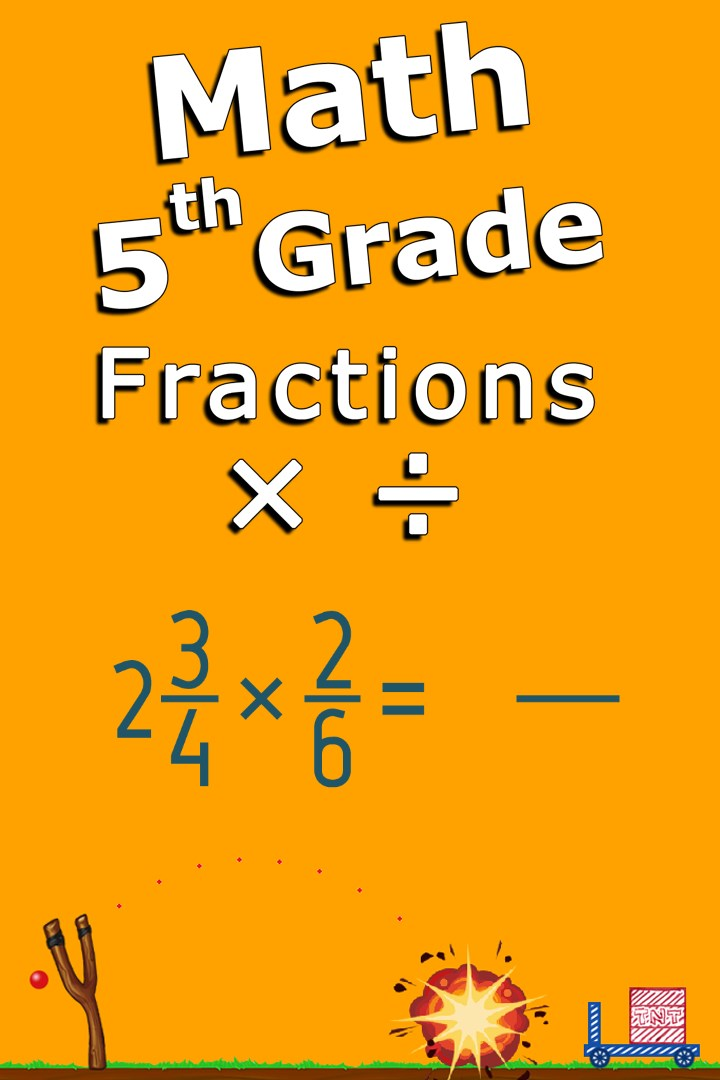Boðið er upp á ókeypis prufutíma fyrir þetta forrit189 kr.
189 kr.
Overview Kerfiskröfur Reviews Related

## Lýsing

Intuitive interface powered by handwriting input and a choice of three fun and engaging mini games in addition to a regular math trainer mode make our app stand out from the crowd of generic math learning apps. With Multiply and divide fractions - 5th grade math you can practice and improve the following math skills: - Multiply fractions by whole numbers - Multiply two fractions - Multiply a mixed number by a whole number - Multiply a mixed number by a fraction - Multiply two mixed numbers - Divide fractions by whole numbers - Divide whole numbers by fractions - Divide two fractions - Divide fractions and mixed numbers Choose between four different modes: - Math Trainer - Math Shot mini-game - Math Gravity mini-game - Math Trick Shot mini-game

## Ítarlegri upplýsingar

Sergey Malugin

Sergey Malugin

10.12.2018

32,36 MB

#### Aldursflokkun

Fyrir 3 ára og eldri

#### Þetta forrit getur

Fá aðgang að öllum skránum þínum, forritum og stýriskrá
Fá aðgang að tengingunni þinni við internetið

#### Uppsetning

Sæktu þetta forrit eftir að hafa skráð þig inn á Microsoft-reikninginn þinn og settu það upp á allt að tíu Windows 10-tækjum.

#### Tungumál stutt

English (United States)

#### Tilkynna þessa vöru

Skráðu þig inn til að tilkynna þetta forrit til Microsoft